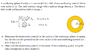# Spherical shells (inner conducting and outer nonconducting)

goohu
Homework Statement:
See picture
Relevant Equations:
V = ## -\int E dl ##
a) I think you find V by just integrating E in regards to R. Then we integrate from the point of interest, which is a, to the 0 potential which is (R = 2a)?

b) If the same logic as a) applies here as well then we should integrate from the point of interest to the 0 potential. This should be from 0 to 2a.

#### Attachments

•Untitled1.png
18.9 KB · Views: 80

Homework Helper
Gold Member
2022 Award
I think you find V by just integrating E in regards to R
Yes, except that R is a given constant, so better to say wrt some new variable, r say.
b) If the same logic as a) applies here as well then we should integrate from the point of interest to the 0 potential.
Yes, except that there is an easier way. Hint: it's a conductor.

•goohu
goohu
R is a constant? Can you explain why? E(R) seems to be a function depending on R, for me it seems like its a variable? I don't understand. What do you mean with wrt?

For b) if its a conductor you could use Gauss law I guess. C = Q/V

I'm mostly familiar with using it to determine the capacitance between two conducting plates. Usually you find E(R) then you integrate to find V and integrate from 0 to d , if d is the distance between the plates.

In this case it's from one conducting shell to origin. I'm unsure about the integration limits.
Is it from 0 to a, or a to 0?

Homework Helper
Gold Member
2022 Award
R is a constant?
Sorry, dealing with too many threads at once.

For b, what do you know about the potential within a conductor?

Last edited:
goohu

b)
The electric field inside a conductor (in this case 0 to a) is 0. So V should be a constant. Not sure if we need to go further than this?

I am unsure because of the wording in the task : "using the same assumptions as those of part (a)."
The assumption being "the electrostatic potential on the outer surface of the non-conducting material is 0 V." The stated assumption shouldn't affect the problem?

Homework Helper
Gold Member
b)
The electric field inside a conductor (in this case 0 to a) is 0. So## V## should be a constant. Not sure if we need to go further than this?
Yes, ##V## is constant throughout the conductor. You need to express V at the center of the conductor in terms of ##\varepsilon_0, a## and ##\rho##.

I am unsure because of the wording in the task : "using the same assumptions as those of part (a)."
The assumption being "the electrostatic potential on the outer surface of the non-conducting material is 0 V." The stated assumption shouldn't affect the problem?
The choice of where ##V = 0## does affect the answer to part (b). For part (b) you want to still take ##V=0## at the outer surface of the non-conductor.

goohu
There is the equation for V. I assume both shells has the same charge density (a bit unclear to me).

## V = \int \frac{\rho_v}{4\pi\epsilon_0R} dV = \int \frac{\rho_v}{4\pi\epsilon_0R} R^2 sin\theta dRd\phi d\theta = \frac{\rho_v}{\epsilon_0} \int R dR = \frac{\rho_v a^2}{2\epsilon_0} ## (integrating from 0 to a)

I am unsure where to go from here?

Homework Helper
Gold Member
a) I think you find V by just integrating E in regards to R. Then we integrate from the point of interest, which is a, to the 0 potential which is (R = 2a)?
Yes. What do you get for an answer?

b) If the same logic as a) applies here as well then we should integrate from the point of interest to the 0 potential. This should be from 0 to 2a.
As @haruspex stated, you could do this but there is an easier way. As you stated in post #5, you know that V is constant throughout the conductor. So, how does the potential at the center of the conductor compare to the potential at the surface of the conductor?

goohu
a) we are integrating from a to 2a.
## V = -\int E dl = -\int \frac{R^3 \rho}{3\epsilon_0 R^2} + \int \frac{a^3 \rho}{3\epsilon_0 R^2} = - \frac {\rho}{3 \epsilon_0} \int R dR + \frac{a^3 \rho}{3 \epsilon_0} \int \frac{1}{R^2} dR = -\frac{\rho a^2}{2\epsilon_0} + \frac{a^2 \rho}{6\epsilon_o} ##

b) Let's assume we integrate from 0 to 2a. Shouldnt the answer be the same as a) if the electric field is 0 inside the conductor? This already seems easy enough to me.

But to answer your question, I think it should be the same?

Homework Helper
Gold Member
a) we are integrating from a to 2a.
## V = -\int E dl = -\int \frac{R^3 \rho}{3\epsilon_0 R^2} + \int \frac{a^3 \rho}{3\epsilon_0 R^2} = - \frac {\rho}{3 \epsilon_0} \int R dR + \frac{a^3 \rho}{3 \epsilon_0} \int \frac{1}{R^2} dR = -\frac{\rho a^2}{2\epsilon_0} + \frac{a^2 \rho}{6\epsilon_o} ##

b) Let's assume we integrate from 0 to 2a. Shouldnt the answer be the same as a) if the electric field is 0 inside the conductor? This already seems easy enough to me.

But to answer your question, I think it should be the same?
For part (a) what limits of integration did you use?

Part (b) is really that easy.goohu
for a) integration limits were a to 2a.

Homework Helper
Gold Member
for a) integration limits were a to 2a.
Getting the signs correct can be tricky. Recall that the basic relation is

##V(B) - V(A) = -\int_A^B \mathbf E \cdot d\mathbf l##

If you integrate from ##a## to ##2a##, what does the left-hand side of the above relation become? In particular, which of ##V(A)## and ##V(B)## would be zero and which would be the potential at the point that you are interested in?